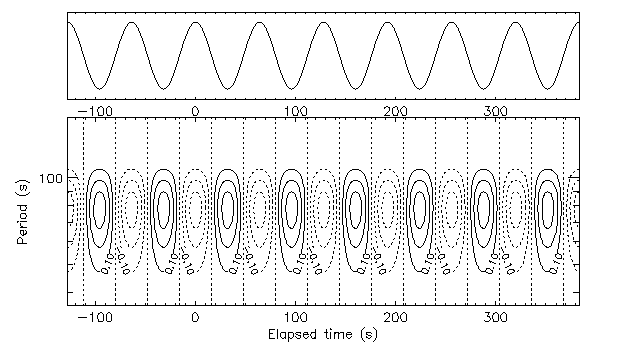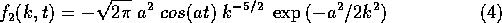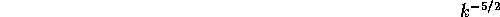Continuous wavelet transformsNext: Interpretation: Up: No Title Previous: Analysis of a

Continuous wavelet transforms

Therefore, we see that some spectral resolution is achieved by selection of the wavelet size, and some temporal resolution follows from the location of the wavelet relative to the signal (Fig. 6).Figure 6: Contour line representation of the wavelet transform for a near-continuum of durations.

We can identify the individual peaks and troughs of the cosine wave as well as their frequency: this compromise between the temporal domain and the spectral domain is the hallmark of wavelet transforms. The cost of this compromise is the need to map the signal as a function of both time and duration.

Analytically:The normalizationfactor is introduced for later convenience. In the case of our cosine function, cos(at) in general, the transform can be calculated exactly, and isfrom which we can see the basic cosine echoing the original signal, the power-lawshowing a steep decrease in magnitude toward larger's (short durations) and the exponential drop-off toward small's (long durations)

Of course, the time () and duration () axis are discretized in actual data processing. The result, a wavelet map, is shown on Fig. 6.Jacques Lewalle
Mon Nov 13 10:51:25 EST 1995